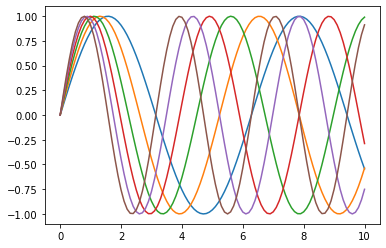# my first ipython notebook post

## my first post with ipynb¶

from matplotlib import pyplot as plt
import numpy as np

x = np.linspace(0,10,101)

fig, ax = plt.subplots()
for i in range(6):
y = np.sin((1+i*0.2)*x)
ax.plot(x, y)#### finished tests¶

this is just some text with some math in it: $x+y = 3 \alpha +\beta$